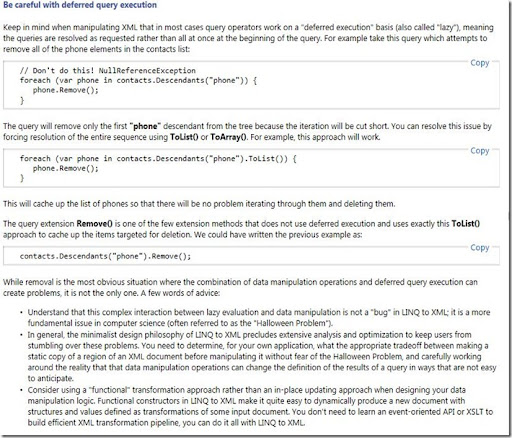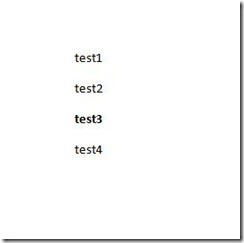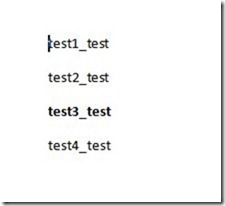## Tuesday, June 28, 2011

### WTF is a Monad by Robert C. Martin in F#

I have read some material describing Computation Expressions and knew computation expressions are the F# implementation of monads, but I did know why one should use a monad.

A few days ago saw the video of presentation by Robert C. Martin (Uncle Bob) at the Norwegian Developers Conference called “WTF is a Monad” and now it makes sense to me.

It is a proven way of solving problems. If you:

• have a problem in one domain
• you can transform it to another domain
• solve the problem in the other domain
• and can transform it back

you have a solution.

I have translated some of the code of the presentation to F#. I tried to stay as close to the original code as possible and did not do much refactoring. I skipped the last two examples (distributions an state).

Update: I did not implement the examples that required lift. I have not figured out how to do that. I have created the lift myself (details: http://bugsquash.blogspot.com/2010/12/notes-on-haskell-functors-and-f.html)

I like to thank Robert for the permission to share the code. Feel free to add improvements in the comments.

`//Non-Monadic dotslet dotsToN (d:string) =    d.Lengthlet result1 = dotsToN "....."let nToDots (n:int) =    new string('.', n)let result2 = nToDots 5let addDots da db =    let a = dotsToN da    let b = dotsToN db    nToDots (a+b)let result3 = addDots "..." "..."//Monadic dotslet dotResult = nToDotslet dotBind d (f:int -> string) =    d |> dotsToN |> flet addDots' da db =    dotBind da (fun a ->        dotBind db (fun b ->            dotResult (a + b)))    let result4= addDots' "..." "..."//dot-Monadtype dotBuilder()=    member x.Bind(d,(f:int -> string)) = d |> dotsToN |> f    member x.Return(n) = new string('.', n)let dot = new dotBuilder()let addDots'' da db =    dot { let! x = da          let! y = db          return x + y        }let result5= addDots'' "..." "..."let multiplyDots da db =    dot { let! x = da          let! y = db          return x*y        }let result6= multiplyDots "..." "..."let dcd dt du =    dot { let! tens = dt          let! units = du          return (10 * tens + units)        }let result7= dcd "..." "....."// In Clojure the monad gets the lift for free// In F# we have to create it ourselves// More info: http://bugsquash.blogspot.com/2010/12/notes-on-haskell-functors-and-f.html --//let liftDots_1 f a1 =     dot {             let! x1 = a1             return f x1         }let liftDots_2 f a1 a2 =     dot {             let! x1 = a1            let! x2 = a2            return f x1 x2        }let liftDots_4 f a1 a2 a3 a4 =     dot {             let! x1 = a1            let! x2 = a2            let! x3 = a3            let! x4 = a4            return f x1 x2 x3 x4        }let substractDots = liftDots_2 (-)let result7a= substractDots "....." ".."let mean4 a b c d = (a+b+c+d)/4let dmean4 = liftDots_4 mean4let result7b= dmean4 "..." ".." "..." "...."////complex number (inspection)//open System.Numericslet c_0 = Complex.Zerolet c_1 = Complex.Onelet c_i = Complex.ImaginaryOnetype complexBuilder()=    member b.Return(x:float) = new Complex(x,0.0)     member b.Bind((c:Complex),(f:float -> Complex)) =         if c.Imaginary = 0.0 then            f c.Real        else             failwithf "Imaginary"   let complex = new complexBuilder()let addComplex c1 c2 =    complex { let! x = c1              let! y = c2              return x + y            }let result8= addComplex c_1 c_1;;let result9= addComplex c_1 c_i;; //System.Exception: Imaginarylet result10= addComplex c_i c_i;; //System.Exception: Imaginary//nulltype noNullBuilder()=    member b.Return(mv) = mv     member b.Bind(mv,f) = if (mv = null) then null else f mvlet nuNull = new noNullBuilder()// int can not be null in .Netlet fragile (a:string) (b:string) (c:string) =    if (a= null||b=null||c=null)    then        "CRASH"    else        a + b + clet result11 = fragile "a" "b" "c"let result12 = fragile "a" null "c" //"CRASH"let safeFragile (a:string) (b:string) (c:string) =    nuNull { let! x = a             let! y = b             let! z = c             return x + y + z            }let result13 = safeFragile "a" "b" "c"let result14 = safeFragile "a" null "c" //val result14 : string = null//Listlet l_a = [1;2;3]let l_b = [4;5;6]let addList = seq {for a in l_a do                    for b in l_b do                     yield a+b                  }|> Seq.toListlet multList = seq {for a in l_a do                    for b in l_b do                     yield a*b                  }|> Seq.toList//helper for the demolet flatten l =     let rec flatten' l result =        match l with         |[] -> result        |h::t -> flatten' t (h@result)    flatten' (l|>List.rev) []let result15 = flatten [[1;2;3];[7;8;9]]type listBuilder()=    member b.Return(v) = [v]    member b.Bind(mv,f) = mv |> List.map f |> flattenlet list = new listBuilder()let addList' a b = list {                       let! x = a                       let! y = b                       return x + y                     }let result16 = addList' l_a l_blet multList' a b = list {                       let! x = a                       let! y = b                       return x * y                     }let result17 = multList' l_a l_b//distribution example see Expert F# of Don Syme page 239..244//state http://fsharpcode.blogspot.com/2008/12/f-state-monad-type-state-state-state-of.html`

## Wednesday, June 22, 2011

### LINQ to XML, when lazy is too lazy in F# (and C# )

Today a F# program did not work as expected. This is a small example I created:

```let xml ="<a><b>1</b><b>2</b></a>"
let doc = XDocument.Parse(xml)

let getXElements_b (doc:XDocument) =
let els = doc.Root.Elements(XName.Get("b"))
els```
```//test
let result1 = doc |> getXElements_b |> Seq.toList
//val result1 : XElement list = [<b>1</b>; <b>2</b>]
//the expected result ```
```
let proces (el:XElement) =
XElement(XName.Get("c")) |> el.ReplaceWith

doc |> getXElements_b |> Seq.iter proces

let result2 =  doc.ToString();;
//val result2 : string = "<a>
//  <c />
//  <b>2</b>
//</a>"
```
This is not the expected result. I expected:

```val result2 : string = "<a>
<c />
<c />
</a>"
```

I can fix it by changing the getXElements_b function:

```let getXElements_b (doc:XDocument) =
let els = doc.Root.Elements(XName.Get("b")) |> Seq.toList
els
```

With this adjustment everything works fine. It seems that I have to force the the computation.

This is a problem in C# too.

```private void GetXml()
{
var xml = "<a><b>1</b><b>2</b></a>";
var doc = XDocument.Parse(xml);
var els = doc.Root.Elements(XName.Get("b"));

foreach (var el in els)
{
el.ReplaceWith(new XElement(XName.Get("c")));
}

txtResult.Text = doc.ToString();
}```
`produces:`

<a>
<c />
<b>2</b>
</a>

When I step with the debuger through the code the foreach is only one time triggered.

With support of Google I found the following document:

http://msdn.microsoft.com/en-us/library/bb308960.aspxYou can learn something new everyday.

The "Halloween Problem" for XML APIs:

Query Composition using Functional Programming Techniques in C# 3.0:

## Monday, June 20, 2011

### Update an open XML word document in F#

` `
`I have created a small function in F# to update the content of a word document.`
```//reference to the package
#r "WindowsBase"
//reference to the Xml and Linq
#r "System.Xml"
#r "System.Xml.Linq"

open System
open System.IO
open System.IO.Packaging
open System.Xml
open System.Xml.Linq

//helper to create XName with a namespace
let xname str =
XName.Get(str, "http://schemas.openxmlformats.org/wordprocessingml/2006/main")

// the main function
// changeDoc copies a word .docx document and updates the content by changing the xml that represents the content
let changeDoc oldPath newPath (updateDocument:XDocument -> XDocument) =
//update the package part
let updatePart (part:PackagePart) =
using (part.GetStream(FileMode.Open, FileAccess.Read)) (fun stream ->
let xDocumentOut = stream |> XDocument.Load |> updateDocument
xDocumentOut.Save(part.GetStream(FileMode.Create, FileAccess.Write))
)

File.Copy(oldPath, newPath, true)
using (Package.Open(newPath, FileMode.Open, FileAccess.ReadWrite)) (fun package ->
let uri = new Uri("/word/document.xml", UriKind.Relative )

uri |> package.GetPart|> updatePart
)

// test function to update the document
// adds "_test" to every text  in the document.
let updateText (xDoc:XDocument) =
"t" |> xname  |> xDoc.Descendants |> Seq.iter (fun x -> x.Value <- x.Value + "_test")
xDoc

//test
changeDoc @"D:\Tmp\test.docx" @"D:\Tmp\test_copy.docx" updateText;;
```
`Before "D:\Tmp\test.docx":`
``
` `
`After "D:\Tmp\test_copy.docx":`
``

## Wednesday, June 8, 2011

### Creation of a very simple F# model of a spreadsheet

Today I posted  a F# snipped at http://fssnip.net/5F.

It creates an Open Xml spreadsheet and does not depend on the Open Xml SDK.

To add data to the sheet you need SheetData . I have created a small model op a spreadsheet and some functions to generate the SheetData.

First the model:
// column * value
type Cell =
| Text of string * string
| Number of string * int
| Formula of string * string

// row number * cells in the row
type Row =
|Cells of int * Cell list

//list of rows
type Sheet =
| Rows of Row list

Notice that the model reflects the structure of the Open Xml specifications. Sheets contain Rows and Rows contain Cells.  I have modeled three types of cells inline text, numbers and formulas. There are more. From the specifications (17.18.11 ST_CellType (Cell Type)):
 Enumeration Value Description b (Boolean) Cell containing a boolean. d (Date) Cell contains a date in the ISO 8601 format. e (Error) Cell containing an error. inlineStr (Inline String) Cell containing an (inline) rich string, i.e., one not in the shared string table. If this cell type is used, then the cell value is in the is element rather than the v element in the cell (c element). n (Number) Cell containing a number. s (Shared String) Cell containing a shared string. str (String) Cell containing a formula string.

These are the tests:
`//test`
`let cell1 = Text("A","test1")`
`let cell2 = Number("B", 42)`
`let cell3 = Text("A","test2")`
`let cell4 = Number("B", 43)`
`let cell5 = Formula("B", "SUM(B1:B2)")`
` `
`let row1 = Cells(1, [cell1; cell2])`
`let row2 = Cells(2, [cell3; cell4])`
`let row3 = Cells(3, [cell5])`
` `
`let sheet = Rows([row1; row2; row3])`

This are the required functions to generate the SheetData:
`let createTextCell column rowNumber (value:string) =`
`    let cell = new XElement(xname "c",`
`                                new XAttribute(xnameEmpty "r", column + rowNumber.ToString()),`
`                                new XAttribute(xnameEmpty "t", "inlineStr"),`
`                                    new XElement(xname "is",`
`                                        new XElement(xname "t", value)))`
`    cell`
` `
`//test`
`let result1 = createTextCell "A" 1 "abc"`
` `
`let createNumberCell column rowNumber (value:int) =`
`    let cell =new XElement(xname "c",`
`                                new XAttribute(xnameEmpty "r", column + rowNumber.ToString()),`
`                                new XAttribute(xnameEmpty "t", "n"),`
`                                    new XElement(xname "v", value`
`                                        ))`
`    cell`
` `
`let createFormulaCell column rowNumber (formula:string) =`
`    let cell =new XElement(xname "c",`
`                                new XAttribute(xnameEmpty "r", column + rowNumber.ToString()),`
`                                new XAttribute(xnameEmpty "t", "n"),`
`                                    new XElement(xname "f", formula`
`                                        ))`
`    cell`
` `
`//test`
`let result2 = createNumberCell "B" 1 4`
` `
`let createCell rowNumber = function`
`    | Text (culumn, value) -> createTextCell culumn rowNumber value`
`    | Number (culumn, value) -> createNumberCell culumn rowNumber value`
`    | Formula (culumn, formula) -> createFormulaCell culumn rowNumber formula`
` `
`//test`
`let result3 =createCell 1 cell1`
`let result4 =createCell 1 cell2`
` `
`let createRow = function`
`    |Cells (rowNumber, cells) -> `
`        let rowElement = new XElement(xname "row", new XAttribute(xnameEmpty "r", rowNumber))`
`        cells |> List.map (createCell rowNumber) |> rowElement.Add`
`        rowElement`
` `
`let result5 = createRow row2`
` `
`let createSheetData (sheet:Sheet) =`
`    let createRows =  function`
`        | Rows rows -> rows|> List.map createRow`
`    let sheetData = new XElement(xname "sheetData")`
`    sheet|> createRows |> sheetData.Add`
`    sheetData`
` `
`let result6 = createSheetData sheet`

This is the result:

Because the sheet contains a formula in cell B3 Excel will calculates the result.

## Tuesday, June 7, 2011

### Create Open XML Word document in F# (update)

This time I will create a word document (open xml) in F# without using the Open XML SDK and using XML libraries. Things have been intentionally kept simple.

I start by modelling a simple word document:

There are Two types of paragraphs: normal and bold ones:
1. //reference to the package
2. #r "WindowsBase"
3.
4. open System
5. open System.IO
6. open System.IO.Packaging
7.
8. type Paragraph =
9.     | Normal of string
10.     | Bold of string

And a document is a list of paragraphs:
1. type Document =
2.     | Paragraphs of Paragraph list

This is the test:
1. //test
2. let list = [Normal("test1"); Normal("test2"); Bold("test3"); Normal("test4")]
3. let doc = Paragraphs(list)

Next we have the to create an xml version of document:

1. //helpers

2.

4. let encodedXml (text: string) =

5.     text.Replace("&", "&amp;").Replace("<", "&lt;").Replace(">", "&gt;").Replace("\"", "&quot;").Replace("'", "&apos;")

6.

7. let xml1 = "<node>it's my \"node\" & i like it<node>"

8. let encode = encodedXml xml1

9.

10. let addString acc item = acc + "\r\t" + item

11.

12. let createParagraphNormal text =

13.     "<w:p>

14.       <w:r>

15.         <w:t>" + encodedXml text +

16.         "</w:t>

17.       </w:r>

18.     </w:p>"

19.

20. let createParagraphBold text =

21.     "<w:p>

22.       <w:r>

23.         <w:rPr>

24.          <w:b />

25.         </w:rPr>

26.         <w:t>" + encodedXml text +

27.         "</w:t>

28.       </w:r>

29.     </w:p>"

30.

31. let createParagraph = function

32.     | Normal(text) -> createParagraphNormal text

33.     | Bold (text) -> createParagraphBold text

34.

35. let createParagraphs = function

36.     | Paragraphs(list) -> list|> List.map createParagraph |> List.reduce addString

37.

38. //test

39. let p = createParagraphs doc

40.

41. let createDocument doc =

42.     let startDocument = @"<?xml version=""1.0"" encoding=""utf-8""?>

43.                     <w:document xmlns:w=""http://schemas.openxmlformats.org/wordprocessingml/2006/main"">

44.                       <w:body>"

45.     let endDocument = "  </w:body>

46.                         </w:document>"

47.     let content = createParagraphs doc

48.     startDocument + content + endDocument

Then we have to create a package, add the document and save it to disk:

1. //add the document to package and save

2. let createFile doc (fileName:string) =

3.     using (Package.Open(fileName, FileMode.Create, FileAccess.ReadWrite))(fun package ->

4.         let uri = new Uri("/word/document.xml", UriKind.Relative )

5.         let partDocumentXML =

6.             package.CreatePart(

7.                                 uri,

8.                                 "application/vnd.openxmlformats-officedocument.wordprocessingml.document.main+xml" )

9.

10.         using(new StreamWriter(partDocumentXML.GetStream(FileMode.Create, FileAccess.Write)))(fun stream ->

11.             doc |> createDocument |> stream.Write

12.         )

13.

14.         package.CreateRelationship(

15.                                     uri,

16.                                     TargetMode.Internal,

17.                                     "http://schemas.openxmlformats.org/officeDocument/2006/relationships/officeDocument",

18.                                     "rId1") |> ignore

19.     )

20.

21. //test

22. let fileName = @"D:\Tmp\test.docx"

23.

24. createFile doc fileName;;

` `
`This is the result:`

` `

I have created a map from twitter to my document model

1. type UserStatus =

3.       ProfileImage : string;

4.       Status : string;

5.       StatusDate : DateTime }

6.

7. let mapUserStatus (us:UserStatus) =

8.     let date = "Date: " + us.StatusDate.ToShortDateString()  + " time: " + us.StatusDate.ToLongTimeString()

10.

11. let addUserStatus acc item = item @ acc

12.

13. let result = xml|> parseTweetXml |> List.map mapUserStatus|> List.reduce addUserStatus

This results in the following document:

## Monday, June 6, 2011

### Create Open XML Spreadsheet in F# (update)

I have updated the code snippet for the creation of a spreadsheet in F#. I rewritten it in a more functional way an I have added an test with data.

1: //reference to the Open Office SDK
2: #r @"C:\Program Files (x86)\Open XML SDK\V2.0\lib\DocumentFormat.OpenXml.dll"
3: //reference to the package
4: #r "WindowsBase"
5:
6: open DocumentFormat.OpenXml
7: open DocumentFormat.OpenXml.Packaging
9:
10: let createSpreadsheet (filepath:string) (sheetName:string) (sheetData:SheetData) =
11:     // Create a spreadsheet document by supplying the filepath.
12:     // By default, AutoSave = true, Editable = true, and Type = xlsx.
13:
15:
16:     // Add a WorkbookPart to the document.
18:
19:     // Add a WorksheetPart to the WorkbookPart.
20:     // http://stackoverflow.com/questions/5702939/unable-to-append-a-sheet-using-openxml-with-f-fsharp
22:
23:     worksheetPart.Worksheet <- new Worksheet(sheetData:> OpenXmlElement)
24:
25:     // Add Sheets to the Workbook.
26:     let sheets = spreadsheetDocument.WorkbookPart.Workbook.AppendChild<Sheets>(new Sheets())
27:
28:     // Append a new worksheet and associate it with the workbook.
29:     let sheet = new Sheet(  Id =  StringValue(spreadsheetDocument.WorkbookPart.GetIdOfPart(worksheetPart)),
30:                             SheetId =  UInt32Value(1u),
31:                             Name = StringValue(sheetName)
32:                             )
33:     [sheet :> OpenXmlElement] |> sheets.Append
34:     )
35:
36: //helpers
37: let createCellReference (header:string) (index:int) =
39:
40: let createNumberCell number (header:string) (index:int) =
41:     let cell = new Cell(DataType = EnumValue(CellValues.Number), CellReference = createCellReference header index)
42:     let value = new CellValue(Text = number.ToString())
43:     value |> cell.AppendChild|> ignore
44:     cell :> OpenXmlElement
45:
46: let createTextCell text (header:string) (index:int) =
47:     let cell = new Cell(DataType = EnumValue(CellValues.InlineString), CellReference = createCellReference header index)
48:     let inlineString = new InlineString()
49:     let t = new Text(Text = text)
50:     t |> inlineString.AppendChild |> ignore
51:     inlineString |> cell.AppendChild|> ignore
52:     cell :> OpenXmlElement
53:
54: let createContentRow (text, (number1:int), (number2:int), (index:int)) =
55:     let row = new Row(RowIndex = UInt32Value(uint32(index)))
56:     let cell1 = createTextCell text "A" index
57:     let cell2 = createNumberCell number1 "B" index
58:     let cell3 = createNumberCell number2 "C" index
59:     cell1 |> row.Append
60:     cell2 |> row.Append
61:     cell3 |> row.Append
62:     row :> OpenXmlElement
63:
64: //test
65: let createTestSheetData =
66:     let sheetData = new SheetData()
67:     ("test1", 123, 456, 1) |> createContentRow |> sheetData.AppendChild |> ignore
68:     ("test2", 35, 1231, 2) |> createContentRow |> sheetData.AppendChild |> ignore
69:     ("test3", 345, 21, 3) |> createContentRow |> sheetData.AppendChild |> ignore
70:     sheetData
71:
72: let testData = createTestSheetData
73: let result = createSpreadsheet @"D:\Tmp\test.xlsx" "test" testData;;
74:
75: